# Basic GLSL Displacement Shader in Three.js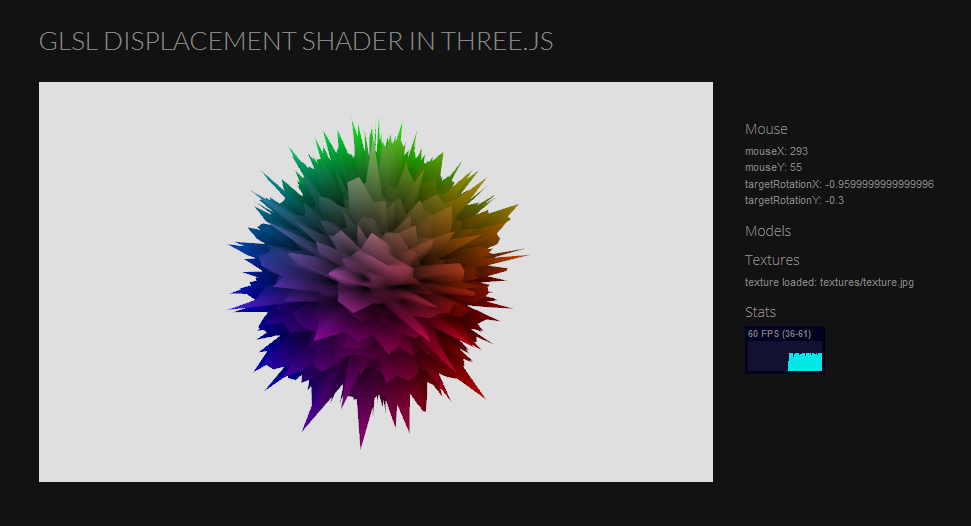This tutorial introduces construction a basic GLSL Displacement Shader in Three.js. It can really apply to any GLSL program, but we’ll take advantage of the three.js ability of using custom shaders. If you’re very new to Three.js, please see Creating a Basic Scene in Three.js

If you’re confident with the basics you can download the full example files for this tutorial.

### A Quick Look At the Shader

If you’ve downloaded the example files, open scene.html and look at line 40.

`<script src="js/shaders/DisplacementShader.js"></script>`

This references our shader script, located in js > shaders > DisplacementShader.js. Taking a peek at the file shows it’s just a javascript object. The two arrays: vertexShader and fragmentShader are used to feed the GLSL code into the WebGL driver to be compiled. The “uniforms” are variables that the vertex and fragment shader will read from. This is all packaged into THREE.DisplacementShader that we can reference in our code.

```THREE.DisplacementShader = {

uniforms: {
texture1: { type: "t", value: null },
scale: { type: "f", value: 1.0 },
},

"varying vec2 vUv;",
"varying float noise;",
"varying vec3 fNormal;",
"uniform sampler2D texture1;",
"uniform float scale;",

"void main() {",

"vUv = uv;",
"fNormal = normal;",

"vec4 noiseTex = texture2D( texture1, vUv );",

"noise = noiseTex.r;",
//adding the normal scales it outward
//(normal scale equals sphere diameter)
"vec3 newPosition = position + normal * noise * scale;",

"gl_Position = projectionMatrix * modelViewMatrix * vec4( newPosition, 1.0 );",

"}"

].join("\n"),

"varying vec2 vUv;",
"varying float noise;",
"varying vec3 fNormal;",

"void main( void ) {",

// compose the colour using the normals then
// whatever is heightened by the noise is lighter
"gl_FragColor = vec4( fNormal * noise, 1. );",

"}"

].join("\n")

};
```

### The Geometry

Open scene.js and find this on line 85

```material = new CustomMat("textures/texture.jpg", THREE.DisplacementShader);
geometry = new THREE.SphereGeometry(1, 80, 80);
mesh = new THREE.Mesh(geometry, material);

As you can see, we add a material, define a basic primitive as our geometry.? We then bind them together into a mesh and add it the the group (later added to the scene in the code). CustomMat is an object we’ve created just to enclose our material. The reason we do this is so that we can add different kinds of materials down the road without it being too messy and cluttering the main file. First,?CustomMat accepts the path where the texture to load into the shader is located, then the? actual shader (talked about above).

Let’s now see what CustomMat does to create the material…

### Create the Material

Open OnepixMaterial.js

```var CustomMat = function (texturePath, shader) {

uniforms["texture1"].value = texture;

}

// texture - texture must not be in same folder or there is an error.
var texture = THREE.ImageUtils.loadTexture(path, {}, function () {
\$('#debug #textures').append("texture loaded: " + path + "<br>");
},
function () {
\$('#debug #textures').append("unable to load: " + path + "<br>");
});

return texture;
}
```

CustomMat accepts the texture path and shader we depicted. It then loads the texture using the onepixLoadTexture function. All this function does is load it using THREE.ImageUtils with the added callbacks for success or failure to feed to some debugging text.

The line:

`THREE.UniformsUtils.clone(shader.uniforms);`

takes the uniforms we talked about in the shader file and copies them for manipulation here. You don’t have to include your uniforms in the shader it’s self, you can create them here but I like to do so in the shader to keep things tidy.

Next:

`uniforms[ "texture1" ].value = texture;`

Assigns our loaded texture to the “texture1” uniform for use in the shader.

We then insert the parameters from our shader into THREE.ShaderMaterial for creation.

### A Basic GLSL Shader First

Now for the fun part, lets’ take a closer look at the shader file.

Shaders are great for getting something really cool fairly quickly, but they can be difficult to debug and understand, so we’ll start by making this as simple as possible to begin with by hooking up the shader: js > shaders > SimpleShader.js. (If you’re already pretty good with shaders your can skip to the next step).

To do this, in scene.js simply switch:

`material = new CustomMat("textures/texture.jpg", THREE.DisplacementShader);`

to:

`material = new CustomMat("textures/texture.jpg", THREE.SimpleShader);`

You should new see something like this: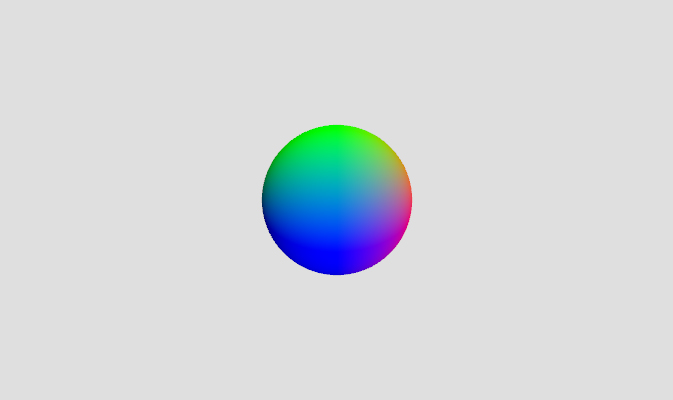```THREE.SimpleShader = {

uniforms: {
texture1: { type: "t", value: null },
scale: { type: "f", value: 1.0 },
},

"varying vec3 fNormal;",

"void main() {",
"fNormal = normal;",
"gl_Position = projectionMatrix * modelViewMatrix * vec4( position, 1.0 );",
"}"

].join("\n"),

"varying vec3 fNormal;",

"void main( void ) {",
// compose the colour using the normals then
// whatever is heightened by the noise is lighter
"gl_FragColor = vec4( fNormal, 1. );",
"}"

].join("\n")

};
```

The uniforms are just there for convenience sake, so we can switch back to the displacement shader without breaking anything. They’re not actually put to use here. “vec3 fNormal” is a varying variable. We must define it in both the vertex and fragment shaders. It’s purpose here is to carry the value copied from the attribute “normal” to the fragment shader. I should mention that every single vertex in the geometry is passed to the vertex shader then to the fragment shader one at a time, so think of shaders as code that is run per vertex.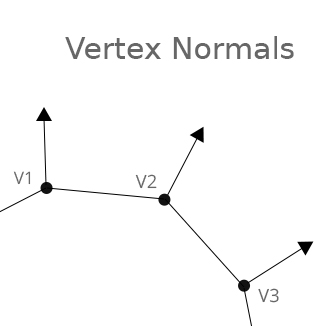The normal attribute is stored in each vertex as a vector that goes from the center of the sphere to it’s diameter. It’s commonly used in shaders to calculate things like lighting where the outward facing direction of vertices are needed.

Then this classic line of code

`gl_Position = projectionMatrix * modelViewMatrix * vec4( position, 1.0 );`

converts the position of the vertex in world space to model space to camera space and finally to the finished 2d product we see on the screen by multiplying matrices.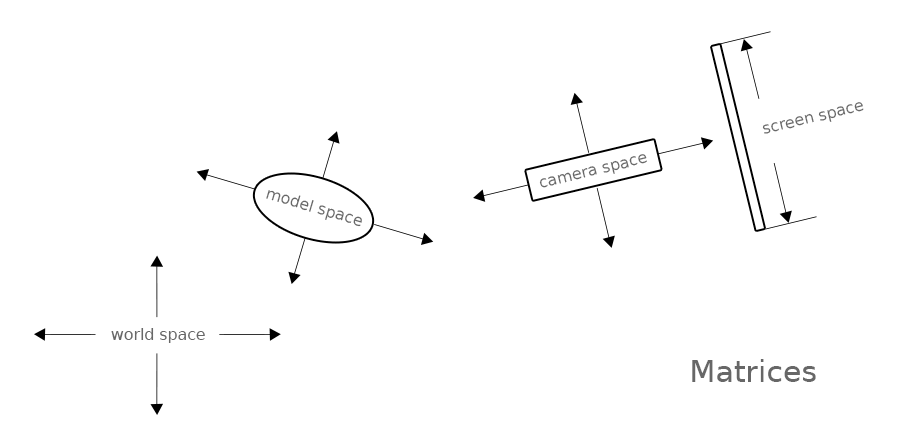It translates it’s space from the point of view of different worlds, where the center of each world is it’s origin. If you’re familiar with 3d modelling software, this would be the origin (world space), the model pivot (model space), the camera pivot (camera space), the screen (the 2d render from the camera).

Our fNormal varying variable (that stores the normal) is then passed to the fragment shader where it’s interpolated. See the below diagram to illustrate interpolation.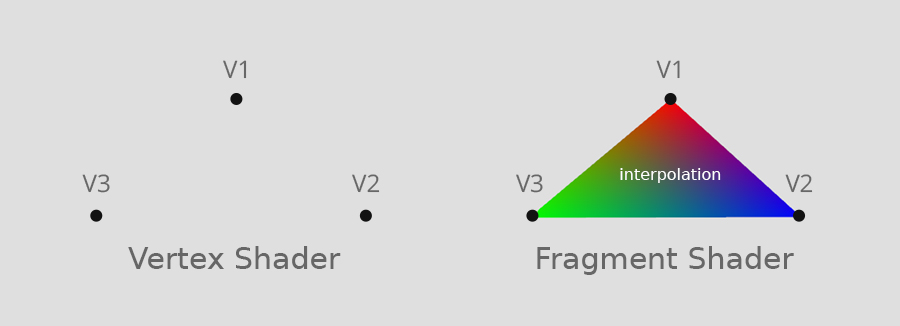It basically takes values passed from the vertex shader and blends them across to the next one creating values in between them for each fragment. The colors here are just for example purposes, they have nothing to do with the resemblance to our rendered sphere.

Lastly,

`gl_FragColor = vec4( fNormal, 1. );`

outputs the final color of the interpolated fragment. The vec4 is for storing rgba color values. Since we’ve inserted our normal vectors into the rgb you’ll see now why our sphere is red on one side, blue on the other and green on top. The 1 is for the color’s alpha which is opaque in this case.

Just before moving onto the final shader, change this line:

`gl_Position = projectionMatrix * modelViewMatrix * vec4( position, 1.0 );`

to this:

`gl_Position = projectionMatrix * modelViewMatrix * vec4( position + normal, 1.0 );`

The sphere should now be twice as big!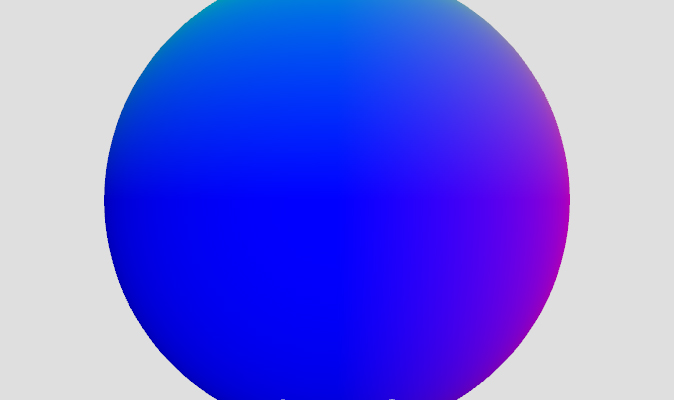adding the normal vector to the vertex position scales the vertices along it’s normal (the outward pointing vector as we recall).

Okay, let’s move on.

First we’ll have to switch:

`material = new CustomMat("textures/texture.jpg", THREE.SimpleShader);`

Back to:

`material = new CustomMat("textures/texture.jpg", THREE.DisplacementShader);`

And again looking at the code in js > shaders > DisplacementShader.js

```THREE.DisplacementShader = {

uniforms: {
texture1: { type: "t", value: null },
scale: { type: "f", value: 1.0 },
},

"varying vec2 vUv;",
"varying float noise;",
"varying vec3 fNormal;",
"uniform sampler2D texture1;",
"uniform float scale;",

"void main() {",

"vUv = uv;",
"fNormal = normal;",

"vec4 noiseTex = texture2D( texture1, vUv );",

"noise = noiseTex.r;",
//adding the normal scales it outward
//(normal scale equals sphere diameter)
"vec3 newPosition = position + normal * noise * scale;",

"gl_Position = projectionMatrix * modelViewMatrix * vec4( newPosition, 1.0 );",

"}"

].join("\n"),

"varying vec2 vUv;",
"varying float noise;",
"varying vec3 fNormal;",

"void main( void ) {",

// compose the colour using the normals then
// whatever is heightened by the noise is lighter
"gl_FragColor = vec4( fNormal * noise, 1. );",

"}"

].join("\n")

};
```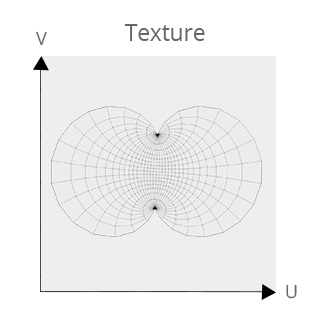This time we’ll be using the uniforms texture1 and scale. “texture1” holds the texture data that was fed into the shader from the CustomMat function. Then the? “texture2D”? sampler is used to read the current texel based on the current UV co-ordinate of the vertex. Think of uvs as flattened 2d representations of the geometry. UVS are often created when exported from a 3d program.

To simplify things, noiseTex.r is used indicating that we only want to take the red values from the texture. This is because we only need the texture’s light and dark areas to make height information.

The line:

`vec3 newPosition = position + normal * noise * scale;`

protrudes the vertices outward based on the noise value. As we fount in the previous step, adding the normal vector protrudes the vertices out. The scale uniform is multiplied in as a way you can control the height of the extrusion.

Lastly, in the fragmentshader the only difference between this and the SimpleShader is that noise is multiplied into the color to make darkness in the low areas and lighter in the high ones.

`gl_FragColor = vec4( fNormal * noise, 1. );`

You could add time as a uniform and cause the bumps to animate or provide noise in the fragment shader to make a more detailed, smooth deformation, but this is a start.

I hope this help you to have a basic understanding of custom GLSL shaders in three.js as well as displacement mapping which you can apply to any of your GLSL (or HLSL with a little tweaking) applications.